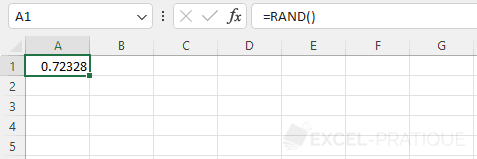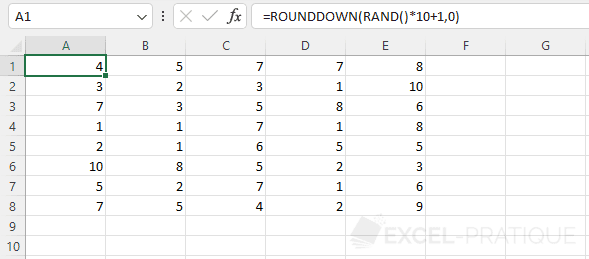# Excel Function: RAND

The Excel function RAND generates a random number greater than or equal to 0 and less than 1.

Usage:

`=RAND()`

## Usage examples

Using the function alone generates a number from 0 to 1 (excluding 1):

``=RAND()``By using RAND and a rounding function, it is also possible to generate whole numbers.

For example, to get a random integer between 1 and 10, use the following formula:

``=ROUNDDOWN(RAND()*10+1,0)``If you want to generate an integer between 1 and 50, simply replace 10 with 50 ...